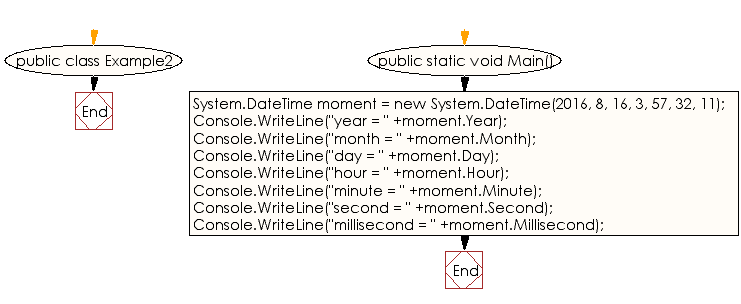﻿ C# - Demonstrate the Day property - w3resource# C# Sharp Exercises: Demonstrate the Day property

## C# Sharp DateTime: Exercise-2 with Solution

Write a C# Sharp program to display the Day properties (year, month, day, hour, minute, second, millisecond etc.)

Sample Solution:-

C# Sharp Code:

``````using System;

public class Example2
{
public static void Main()
{
System.DateTime moment = new System.DateTime(2016, 8, 16, 3, 57, 32, 11);

Console.WriteLine("year = " +moment.Year);

Console.WriteLine("month = " +moment.Month);

Console.WriteLine("day = " +moment.Day);

Console.WriteLine("hour = " +moment.Hour);

Console.WriteLine("minute = " +moment.Minute);

Console.WriteLine("second = " +moment.Second);

Console.WriteLine("millisecond = " +moment.Millisecond);

}
}
```
```

Sample Output:

```year = 2016
month = 8
day = 16
hour = 3
minute = 57
second = 32
millisecond = 11
```

Flowchart :C# Sharp Code Editor:

Improve this sample solution and post your code through Disqus

What is the difficulty level of this exercise?

Test your Programming skills with w3resource's quiz.

﻿